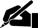Term Paper: Earthquakes Charles Richter Determined That the Seismic

Pages: 1 (328 words)  ·  Bibliography Sources: 1+  ·  Level: College Senior  ·  Topic: Geography  ·  Buy This Paper

Earthquakes

Charles Richter determined that the seismic waves emitted from an earthquake could be used to estimate its magnitude, and he developed a calibrated system for measuring these waves commonly known as the Richter Scale (Earthquakes). The Richter Scale formula is based on the discovery that the larger the earthquake, the larger the amplitude of ground motion at a given distance from the quake. The Richter Scale equation is:

R (x) =.67 • log (.37x) + 1.46

R (x) equals the magnitude of that earthquake on the Richter scale and x equals the energy of an earthquake in kilowatt-hours (Earthquakes). The small numeric differences in values of R (x) can be misleading if one does not understand that the scale is logarithmic, which means that each whole number of the scale actually increases by 10. Thus, a magnitude 7.0 earthquake is ten times stronger than a 6.0; a 7.0 is 100 times greater than a 5.0; and a 7.0 is 1000 times greater than… [END OF PREVIEW]

Ordering Options:

or

2.  Let us write a NEW paper for you!Most popular!

Charles Horton Cooley Term Paper…

Cite This Term Paper:

APA Format

Earthquakes Charles Richter Determined That the Seismic.  (2006, May 21).  Retrieved October 24, 2019, from https://www.essaytown.com/subjects/paper/earthquakes-charles-richter-determined/2149440

MLA Format

"Earthquakes Charles Richter Determined That the Seismic."  21 May 2006.  Web.  24 October 2019. <https://www.essaytown.com/subjects/paper/earthquakes-charles-richter-determined/2149440>.

Chicago Format

"Earthquakes Charles Richter Determined That the Seismic."  Essaytown.com.  May 21, 2006.  Accessed October 24, 2019.
https://www.essaytown.com/subjects/paper/earthquakes-charles-richter-determined/2149440.